### Home > CALC > Chapter 10 > Lesson 10.1.6 > Problem10-53

10-53.
1. Decide if the following series converge or diverge. Justify your answer, including which test you used to determine your answer. Homework Help ✎

1.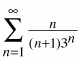2.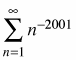3.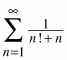4.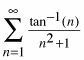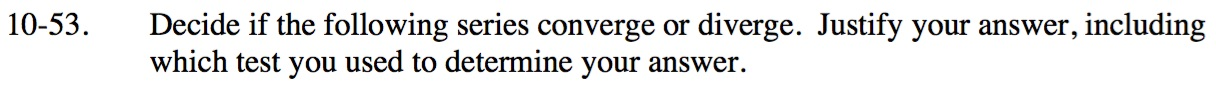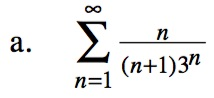$\text{Compare this series to }\sum_{n=1}^\infty \frac{1}{3^n}.$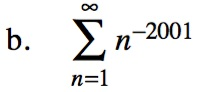This is a p-Series.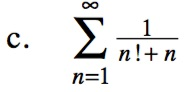$\text{Compare this series to }\sum_{n=1}^\infty \frac{1}{n^2}.$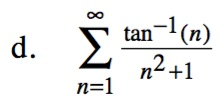$\left|\tan^{-1}(n)\right|<\frac{\pi}{2}$

$\frac{1}{n^2+1}<\frac{1}{n^2}$